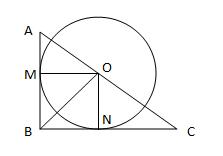#### You may also like### Fitting In

The largest square which fits into a circle is ABCD and EFGH is a square with G and H on the line CD and E and F on the circumference of the circle. Show that AB = 5EF. Similarly the largest equilateral triangle which fits into a circle is LMN and PQR is an equilateral triangle with P and Q on the line LM and R on the circumference of the circle. Show that LM = 3PQ### Look Before You Leap

Can you spot a cunning way to work out the missing length?### Triangle Midpoints

You are only given the three midpoints of the sides of a triangle. How can you construct the original triangle?

# Sitting Pretty

##### Age 14 to 16Challenge Level

We received many good solutions of this question. They either use the properties of the area of triangles or similar triangles to solve the problem.

Emile from CNDL and John from South Island School (Hong Kong) used the area of triangles to solve the problem. Emile writes:$xy/ 2$ is the area of the triangle ABC. You can also divide the triangle in to two triangles AB and CBO with area $rx/2$ and $ry/2$ respectively. Thus, we have
$$xy/2=rx/2+ry/2\;.$$ Thus,
\begin{align} xy&=rx+ry\\ xy&=r(x+y)\\ 1/r&=(x+y)/xy\\ 1/r&=1/x+1/y\;.\\ \end{align}

Rachel, Emily, Samantha and Kri from Millais School, Ciaran from St Patrick's School, Wilson's School Maths Club and Nathanael used similar triangles to solve this problem. This is the solution from Ciaran of St Patrick's school.

AB and BC are tangents to the circle, thus ON and OM are perpendicular to BC and AB. Thus triangle ONC and triangle ABC are similar since they share the same angle C and both have a right angle. $ON = r$ and $NC = y-r.$ Thus,
\begin{align} r/(y-r) &= x/y\\ ry &= xy -rx\\ ry + rx &= xy\\ xy&=r(x+y)\\ 1/r&=(x+y)/xy\\ 1/r&=1/x+1/y \end{align}

and the rest of the solution is the same as Emile's.

Congratulations to all of you who did it correctly.